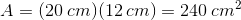## Example Questions

### Example Question #3 : Other Quadrilaterals

Jane plans to re-tile her kitchen with tiles that have a length ofinches and a width ofinches wide. If her kitchen measuresfeet byfeet, how many tiles will she need?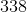Explanation:

When attempting to solve how many pieces of a particular object you need to cover a certain area, always make sure that your units of measurement (for the object and for the area you are covering) are the same. In the case of this question, the units of measurement are different, so you must first convert all measurements to use the same unit.

Since the tiles being used to cover the area are measured in inches, convert all units given to inches:

Length:feet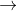inches

Width:feetinches

Then, find the area of the entire space you are covering. Since the space is rectangular (this can be assumed since only length and width are given as measurements), multiply the length and width to find the total area: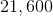square inches.

Next, find the area of each of the tiles: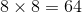square inches. Divide the total area of the space by the area of each of your tiles: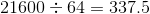. In cases where you are covering a certain area with an object, always round up if you have a decimal (no matter how small), because you cannot simply buyof a tile.

The answer is.

### Example Question #4 : Other Quadrilaterals

Vikram is looking at a scale drawing of a room he is building. The drawing is of a rectangle that measuresinches byinches. If he knows the length of the longer side of the room will measurefeet, what is the area of the room?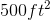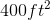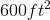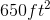Explanation:

When solving such problems, always make sure that your units of measurement are all the same. In the case of this problem, you must convert feet to inches. Multiply 30 by 12 in order to get its equivalent in inches.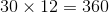We know that the 30 feet corresponds with the side of the drawing that is 18 inches because it is stated that the longest side will be 30 feet. Divide 360 by 18 in order to find the ratio of the actual size of the room to the measurements on the drawing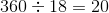This means that the actual measurements of the room are 20 times the measurements of the drawing. Multiply 20 times 12 in order to find the measurement of the shorter side in inches.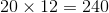Divide both the 360 and 240 by 12 to convert the measurements back into feet.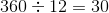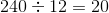Then multiply the results to get the final answer.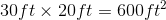### Example Question #5 : Other Quadrilaterals

If a trapezoid has base lengths of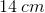and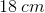and a height of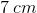, what is its area?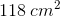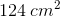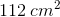Explanation:

Use the formula for area of a trapezoid: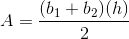Whereis the area,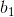and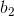are the lengths of the two bases, andis the height.

In this case: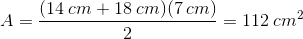### Example Question #6 : Other Quadrilaterals

A parallelogram has a side length of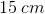, a height of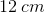, and a base length of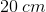. What is its area?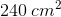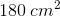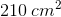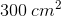Explanation:

Use the formula for area of a parallelogram: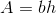Whereis the area,is the base length, andis the height.

In this case: